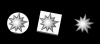# Passing different textures to shader for multiple objetcts

#### Darius Pranskus

##### Member
Hi experts

I am experimenting with the possibility of using normal map textures to enhance the look and feel of my sprites. So I created tree objects - a circle, a square and a star. l of them rotate in Step event.

Also I use a custom shader to use the normal maps, which I prepare it in create event of each object, use it in the DrawBegin event and then reset in DrawEnd event. For example if we take a square object:

Create event
Code:
``````//shader uniforms

//direction light is pointing to, i.e. opposite direction light is coming from.
//light direction is normalized inside vertex shader
light_x = -10;
light_y = 3;
light_z = 50;

//used to keep track of the current texture assigned to shader normal sampler "normal_tex"
//so that we can avoid resetting it unless the texture will change

normal_tex = sprite_get_texture(sprSquareN, 0);``````
DrawBegin event
Code:
``````shader_set(shNormal)
texture_set_stage(normal_tex_uniform, normal_tex);``````
DrawEnd event
Code:
``shader_reset()``
Step event
Code:
``````image_angle += 1;
image_angle = image_angle % 360;
image_alpha = degtorad( image_angle ) / ( 2 * pi );``````

The problem I am facing is that even I pass different textures with texture_set_stage for individual objects, all of my objects use the last normal map texture.My understanding is that texture_set_stage function just sets some stage in the GPU, and because it is the same for each object, that's why it uses it for all of them.

I am still pretty new to GM and shaders, could someone help me what approach should i take to ensure that my shader uses different textures for each object.

I tried to duplicate the shader and use a copy of it on each object, tried to use different names for texture variables but with no luck.

I am evaluating the GMS at the moment and I am about to purchase it if this issue is sorted.

I also uploaded a sample project to illustrate this. Please find it here https://bit.ly/35JEN3K

Thank youy very much

Darius

#### Darius Pranskus

##### Member
Code:
``````//--------------------------------------------------------------
attribute vec3 in_Position;                  // (x,y,z)
//NOTE: alpha is used to encode angle of asteroid.
attribute vec4 in_Colour;                    // (r,g,b,a)
attribute vec2 in_TextureCoord;              // (u,v)
//--------------------------------------------------------------
varying vec2 v_vTexcoord;
varying vec3 v_vColour;
varying vec3 light_dir_transformed;
//--------------------------------------------------------------
uniform vec3 light_dir;  //direction lighting
vec3 light_dir_normalized = normalize( light_dir );  //normalize light direction (change its length to 1)
//--------------------------------------------------------------
void main() {
vec4 object_space_pos = vec4( in_Position.x, in_Position.y, in_Position.z, 1.0);
gl_Position = gm_Matrices[MATRIX_WORLD_VIEW_PROJECTION] * object_space_pos;
//--------------------------------------------------------------
//image_angle of asteroid is encoded into in_Colour alpha component
//note: this means you can't set the alpha of an asteroid by changing image_alpha or by using draw_set_alpha
//0 = 0 degrees
//0.25 = 90 degrees
//0.5 = 180 degrees
//0.75 = 270 degrees
//convert angle to radians by multiplying by 2pi
float angle = in_Colour.a * 6.283185307;
//--------------------------------------------------------------
//build a rotation matrix to transform light_dir into the same "space" as our asteroid
//we do that by rotating light_dir by the opposite direction of our image_angle
//compsition of this rotation matrix...
//    [ cos(angle), -sin(angle) ]
//    [ sin(angle),  cos(angle) ]
vec2 cs = vec2( cos( angle ), sin( angle ) );
mat2 rot = mat2( cs, vec2( -cs.y, cs.x ) );
//--------------------------------------------------------------
//rotate light position opposite direction of image_angle.
//putting rotation matrix on right-hand side
//which will in effect transpose the matrix
//resulting in a rotation in the opposite direction
//than if we put the matrix on the left-hand side
light_dir_transformed = vec3( light_dir_normalized.xy * rot, light_dir_normalized.z );
//--------------------------------------------------------------
//pass texture coordinates to fragment shader
v_vTexcoord = in_TextureCoord;
v_vColour = in_Colour.rgb;
}
//--------------------------------------------------------------``````
Code:
``````//--------------------------------------------------------------
varying vec2 v_vTexcoord;  //(u,v)
varying vec3 v_vColour; //(r,g,b) note: no alpha cuz we're using it for something else
varying vec3 light_dir_transformed;  //(z,y,z)
//--------------------------------------------------------------
uniform sampler2D normal_tex;
//--------------------------------------------------------------
void main() {
//look up normal map colour and remap it to range (-1 to 1)

vec3 normal_colour = texture2D( normal_tex, v_vTexcoord ).rgb * 2.0 - 1.0;

//--------------------------------------------------------------
//compute light level of this fragment.
//am assuming that light_dir_transformed and normal_colour are normalized by this point
//dotting these two vectors will give us the cosine of the angle between them
//0 = the light ans surface vectors point in same direction (full shadow)
//1 = the light and surface vectors point in opposite directions (full light)
//thus if dot( light_dir_transformed, normal_colour ) is 1, then the surface is fully lit at this fragment
float light_level = max(0.4, dot( light_dir_transformed, normal_colour ));
//--------------------------------------------------------------
//look up colour of this fragment
vec4 colour = texture2D( gm_BaseTexture, v_vTexcoord );
//--------------------------------------------------------------
//multiply colour by light level
gl_FragColor = vec4( v_vColour * colour.rgb * light_level, colour.a );
}
//--------------------------------------------------------------``````

#### Azenris

##### Member
draw begin, draw and draw end are not run in that order per instance but all instance draw begins, then all instance draws the all instance ends

•Darius Pranskus and Ido-f

#### Darius Pranskus

##### Member
draw begin, draw and draw end are not run in that order per instance but all instance draw begins, then all instance draws the all instance ends
Thanks, Azenris. This makes sense now.

Could you please advise, how may I approach this to sort it out?

Thank you

Last edited:

#### Darius Pranskus

##### Member
I was able to achieve the desired result by moving the code to draw event
Code:
``````texture_set_stage(normal_tex_uniform, normal_tex);
draw_self();``````
Azenris, thanks again